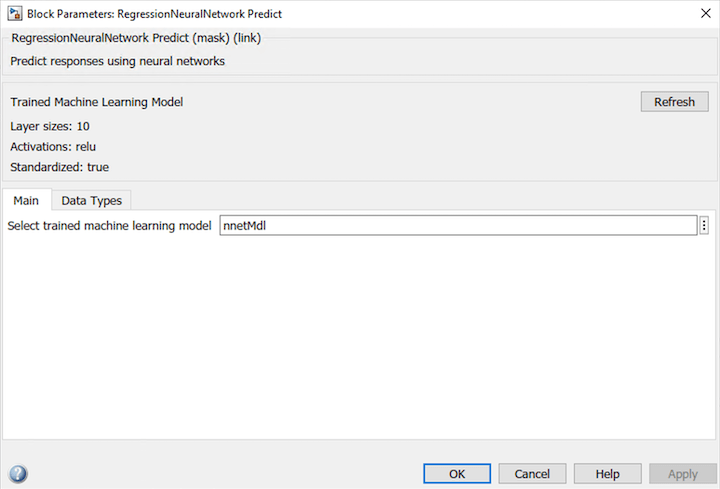# Predict Responses Using RegressionNeuralNetwork Predict Block

This example shows how to use the RegressionNeuralNetwork Predict block for response prediction in Simulink®. The block accepts an observation (predictor data) and returns the predicted response for the observation using the trained neural network regression model.

### Train Regression Model

Load the `cereal` data set. Create the predictor `X` as a numeric matrix that contains 6 features for 77 observations. Create the response `Y` as a numeric vector that contains the calories for each cereal.

```load cereal X = [Carbo Cups Fat Fiber Protein Sugars]; Y = Calories;```

Separate the data into a training set and a test set by using a nonstratified holdout partition. The software reserves approximately 20% of the observations for the test data set and uses the rest of the observations for the training data set.

```rng("default") % For reproducibility of the partition cv = cvpartition(length(Y),"Holdout",0.20);```

Extract the training and test indices.

```trainingInds = training(cv); testInds = test(cv);```

Specify the training and test data sets.

```XTrain = X(trainingInds,:); YTrain = Y(trainingInds); XTest = X(testInds,:); YTest = Y(testInds);```

Train a neural network regression model by passing the training data `XTrain` and `YTrain` to the `fitrnet` function. Specify to standardize the numeric predictors.

`nnetMdl = fitrnet(XTrain,YTrain,"Standardize",true)`
```nnetMdl = RegressionNeuralNetwork ResponseName: 'Y' CategoricalPredictors: [] ResponseTransform: 'none' NumObservations: 62 LayerSizes: 10 Activations: 'relu' OutputLayerActivation: 'none' Solver: 'LBFGS' ConvergenceInfo: [1x1 struct] TrainingHistory: [667x7 table] Properties, Methods ```

`nnetMdl` is a `RegressionNeuralNetwork` model. You can use dot notation to access the properties of `nnetMdl`. For example, you can specify `nnetMdl.TrainingHistory` to get more information about the training history of the neural network model.

This example provides the Simulink model `slexRegressionNeuralNetworkPredictExample.slx`, which includes the RegressionNeuralNetwork Predict block. You can open the Simulink model or create a new model as described in this section.

#### Open Provided Model

Open the Simulink model `slexRegressionNeuralNetworkPredictExample.slx`.

```SimMdlName = 'slexRegressionNeuralNetworkPredictExample'; open_system(SimMdlName)```The `PreLoadFcn` callback function of `slexRegressionNeuralNetworkPredictExample` includes code to load the sample data, train the neural network model, and create an input signal for the Simulink model. If you open the Simulink model, the software runs the code in `PreLoadFcn` before loading the Simulink model. To view the callback function, in the Setup section on the Modeling tab, click Model Settings and select Model Properties. Then, on the Callbacks tab, select the `PreLoadFcn` callback function in the Model callbacks pane.

#### Create New Model

Instead of opening the model provided, you can create a new model. To create a new Simulink model, open the Blank Model template and add the RegressionNeuralNetwork Predict block. Add the Inport and Outport blocks and connect them to the RegressionNeuralNetwork Predict block.

Double-click the RegressionNeuralNetwork Predict block to open the Block Parameters dialog box. You can specify the name of a workspace variable that contains the trained neural network model. The default variable name is `nnetMdl`. Click the Refresh button. The Trained Machine Learning Model section of the dialog box displays the options used to train the model `nnetMdl`.The RegressionNeuralNetwork Predict block expects an observation containing 6 predictor values. Double-click the Inport block, and set the Port dimensions to 6 on the Signal Attributes tab.

Create an input signal in the form of a structure array for the Simulink model. The structure array must contain these fields:

• `time` — The points in time at which the observations enter the model. The orientation must correspond to the observations in the predictor data. In this example, `time` must be a column vector.

• `signals` — A 1-by-1 structure array describing the input data and containing the fields `values` and `dimensions`, where `values` is a matrix of predictor data, and `dimensions` is the number of predictor variables.

Create an appropriate structure array for future predictions.

```cerealInput.time = (0:length(YTest)-1)'; cerealInput.signals(1).values = XTest; cerealInput.signals(1).dimensions = size(XTest,2);```

Import the signal data from the workspace:

• Open the Configuration Parameters dialog box. On the Modeling tab, click Model Settings.

• In the Data Import/Export pane, select the Input check box and enter `cerealInput` in the adjacent text box.

• In the Solver pane, under Simulation time, set Stop time to `cerealInput.time(end)`. Under Solver selection, set Type to `Fixed-step`, and set Solver to `discrete (no continuous states)`.

`sim(SimMdlName);`